# Chapter 11: Vapor-Liquid Separation Processes¶

## Example 11.1-1 Page Number 641¶

In :
#Use of Raoult's Law for Boiling Point Diagram
import numpy as np
from scipy.interpolate import interp1d
from scipy.optimize import root
import scipy.integrate as integrate

#Variable Declaration
T = 95              #Equlibrium temperature in deg C
P = 101.32          #Vapor pressure from table 11.1-1
PA = 155.7          #Vapor pressure of benzene from table 11.1-1 in kPa
PB = 63.3           #Vapor pressure of toulene from table 11.1-1 in kPa
fPT = lambda x:PA*x + PB*(1.-x)-P
sol = root(fPT,0.05)
xA = sol.x
xB = 1.- xA
yA = PA*xA/P
yB = 1.- yA

#Result
print "Liquid and Vapor composions of benzene at 95°C:",round(xA,3),round(yA,3)

Liquid and Vapor composions of benzene at 95°C: 0.411 0.632


## Example 11.2-1 Page Number 642¶

In :
#Equilibrium Contact of Vapor-Liquid Mixture
import numpy as np
from scipy.interpolate import interp1d
from scipy.optimize import root
import matplotlib.pylab as plt

#Variable Declaration
P = 101.32                 #Presure of the vapor in kPa
yA2 = 0.4                  #Mole fraction of benzene
yB2 = 0.6                  #Mole fraction of toulene
V2 = 100.
L0 = 110.
xA0 = 0.3
xB0 = 0.7
xe = np.array([0.000,0.130,0.258,0.411,0.581,0.780,1.000])
ye = np.array([0.000,0.261,0.456,0.632,0.777,0.900,1.000])
#Calculations
#for equimolal overflow
L1 = L0
V1 = V2
fyxe = interp1d(xe,ye, kind='cubic',bounds_error=False)
x = np.arange(0.,1.05,0.05)
y = fyxe(x)
plt.plot(x,y,'r-',xe,xe,'k-')
x1 = 0.4
y1 = (L0*xA0 +V2*yA2 - L1*x1)/V1
x2 = 0.2
y2 = (L0*xA0 +V2*yA2 - L1*x2)/V1
plt.plot([x1,x2],[y1,y2],'k-')
f = lambda x: L0*xA0 +V2*yA2 - L1*x - V1*fyxe(x)
sol = root(f,0.1)
xA1 = sol.x
yA1 = fyxe(xA1)
plt.grid(True)
plt.xlabel('Liquid phase mole fraction, xA')
plt.ylabel('Vapor phase mole fraction, yA')
plt.plot(xA1,yA1,'ro')

#Results
print "Equilibrium compositions of liquid and vapor are:",round(xA1,4),round(yA1,4)

Equilibrium compositions of liquid and vapor are: 0.254 0.4506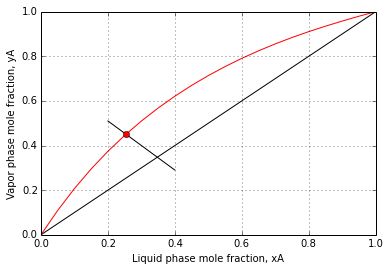## Example 11.3-1 Page Number 645¶

In :
#Relative Volatility for Benzene-Toluene System

#Variable Declaration
PA85 = 116.9              #Pressure of benzene at 85 deg C
PB85 = 46.0               #Pressure of tuolene  at 85 deg C

PA = 204.2                #Pressure of benzene at 105 deg C
PB = 86.0                 #Pressure of toulene at 105 deg C

#Calculation
alpha85 = PA85/PB85
alpha = PA/PB

#Results
print 'Relative Volatility for Benzene-Toluene at 85°C is %4.2f and at 105°C is %4.2f'%(alpha85,alpha)

Relative Volatility for Benzene-Toluene at 85°C is 2.54 and at 105°C is 2.37


## Example 11.3-2 Page Number 647¶

In :
#Simple Differential Distillation
import numpy as np
import matplotlib.pyplot as plt
from scipy.interpolate import interp1d
from scipy.optimize import root
from scipy.integrate import quad, simps, romberg

#Variable Declaration
L1 = 100                 #Feed, mol
xfnp = 0.5               #Mole fraction of n-pentane
xfnh = 0.5               #Mole fraction of n-heptane
P = 101.3                #Pressure in kPa
V = 40.                  #Distilled moles
x = np.array([0.000,0.059,0.145,0.254,0.398,0.594,0.867,1.000])
y = np.array([0.000,0.271,0.521,0.701,0.836,0.925,0.984,1.000])

#Calculations
L2 = L1-V
lnL1L2 = log(L1/L2)

f1 = interp1d(x,y,kind = 'cubic',bounds_error=False)
xx = np.arange(0.20,.601,0.001)
yy = f1(xx)
ff = 1./(yy-xx)
f = interp1d(xx,ff,bounds_error=False)
def Integrant(x):
return f(x)

er = 12.
ub = 0.5

for j in range(len(xx)):
lb = xx[j+1]
zz = romberg(Integrant, lb, ub)
er = abs(zz-lnL1L2)
if er <=0.005:
xL = xx[j]
break
yav = (L1*xfnp-L2*xL)/V
plt.xlim(0.2,0.55)
plt.ylim(0,3.5)
plt.plot(xx,ff,'k-')
xxx = np.arange(xL,xfnp+0.001,0.001)
yyy = f1(xxx)
fff = 1./(yyy-xxx)
plt.fill_between(xxx,fff,0,color='0.6')
plt.text(0.35,1.0,'Area='+str(round(lnL1L2,3)))
plt.xlabel('$x$')
plt.ylabel('$1/(y-x)$')
plt.grid(True)
#Results
print "Residue concentration : ", round(xL,3)
print "Average Distillate concentration:", round(yav,3)

Residue concentration :  0.276
Average Distillate concentration: 0.836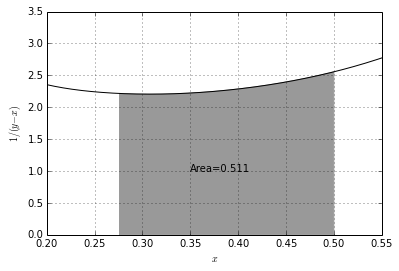## Example 11.4-1 Page Number 656¶

In :
#Rectification of Benzene-Toluene Mixture
import numpy as np
from scipy.interpolate import interp1d
from scipy.optimize import root
import matplotlib.pyplot as plt
from math import ceil

#Variable Declaration
xe = np.array([0.000,0.130,0.258,0.411,0.581,0.780,1.000])
ye = np.array([0.000,0.261,0.456,0.632,0.777,0.900,1.000])

F = 100.               #Feed in kmol/hr
xF = 0.45              #Mole fraction of benzene in feed
xD = 0.95              #Mole fraction of benzene in distillate
xW = 0.10              #Mole fraction of benzene at the bottom
R = 4.0                #Reflux ratio
lambdav = 32099.
Cp = 159.              #Average heat capacity of feed in kJ/kmol.K
Tb = 366.7             #Boiling point of feed (K)
Tf = 327.6             #Temperature of feed (K)

#Calculations

x = np.arange(0.,1.,0.01)
f = interp1d(xe,ye, kind='cubic')
y = f(x)

plt.text(.05, .6, 'Equilibrium Curve')
plt.text(xF,xF-0.1, 'Feed Line')
plt.text(xF+0.05,xF+0.1, 'q-Line')
plt.text(xF+0.3,xF+0.3, 'UO Line')
plt.text(xF-0.2,xF-0.2, 'LO Line')
plt.plot(x,y,'k-')
plt.plot(xD,xD,'ro')
plt.plot(xW,xW,'ro')

plt.annotate('$(x_D,y_D)$', xy=(xD,xD), xytext=(xD,xD-0.02))
plt.annotate('$(x_W,y_W)$', xy=(xW,xW), xytext=(xW,xW-0.02))
plt.xlabel('Liquid mole fraction, x')
plt.ylabel('Vapor mole fraction, y')
plt.plot([0,1.], [0,1.], 'ko-', lw=0.5)

ff = lambda x: f(x)-(mql*x+cql)

plt.plot([0,1,xF,xF],[0,1,xF,0])
a = np.array([[1,1], [xD,xW]])
b = np.array([F,F*xF])
[D,W]= np.linalg.solve(a, b)
q = 1+Cp*(Tb-Tf)/lambdav
muol = R/(R+1)
cuol = xD/(R+1)
mql = q/(q-1.)
cql = xF - mql*xF

plt.plot(xD,xD,'rx')
plt.plot(xW,xW,'rx')
a = np.array([[1,-mql], [1,-muol]])
b = np.array([cql,cuol])
[yi,xi] = np.linalg.solve(a, b)
mlol = (xW-yi)/(xW-xi)
clol = yi - mlol*xi
sol = root(ff,0.52)
xq = sol.x
yq = f(xq)
plt.plot([xF,xq],[xF,yq])
plt.plot([xF,xi],[xF,mql*xi+cql])
plt.plot([xD,xi],[xD,yi])
plt.plot([xW,xi],[xW,yi])
x1 = xD
y1 = xD
n = 0
j = 0
while x1>xW:
y2 = y1
ff = lambda x: y1 -f(x)
sol = root(ff,0.2)
x2 = sol.x
plt.text(x2, y2+0.02, str(n+1))
plt.plot([x1,x2],[y1,y2],'k-')
if x2 > xW:
n = n+1
else:
dxt = x1 - x2
dx = x1 - xW
n = n + dx/dxt
if x2>xW and x2<xi:
j = j + 1
x1 = x2
if x1 <= xi:
c = clol
m = mlol
else:
c = cuol
m = muol
ff = lambda y: x1 - (y - c)/m
sol = root(ff,0.5)
y2 = sol.x
plt.plot([x1,x2],[y1,y2],'k-')

x1 = x2
y1 = y2
nf = n - j
plt.title('McCabe Thiele Diagram')
plt.grid(True)
#Results
print "Rate of distilate",round(D,1),"kmol/hr\nRate of bottoms" ,round(W,1),"kmol/hr"
print 'slope of q-line: %4.2f'%mql
print "Number of equilibrium satges including reboiler for required separation:",round(n,1)
print "Number of equilibrium satges excluding reboiler for required separation:",round(n-1,1)
print 'Feed is introduced on %2d'%(ceil(nf))

Rate of distilate 41.2 kmol/hr
Rate of bottoms 58.8 kmol/hr
slope of q-line: 6.16
Number of equilibrium satges including reboiler for required separation: 7.5
Number of equilibrium satges excluding reboiler for required separation: 6.5
Feed is introduced on  5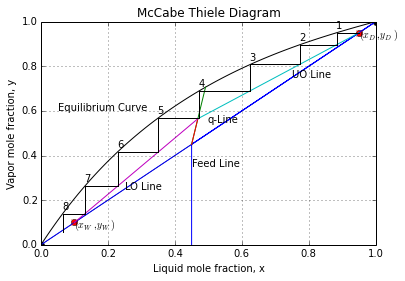## Example 11.4-2 Page Number 660¶

In :
#Minimum Reflux Ratio and Total Reflux in Rectification
import numpy as np
from scipy.interpolate import interp1d
from scipy.optimize import root
import matplotlib.pylab as plt
from math import ceil

#Variable Declaration
xe = np.array([0.000,0.130,0.258,0.411,0.581,0.780,1.000])
ye = np.array([0.000,0.261,0.456,0.632,0.777,0.900,1.000])

F = 100.               #Feed in kmol/hr
xF = 0.45              #Mole fraction of benzene in feed
xD = 0.95              #Mole fraction of benzene in distillate
xW = 0.10              #Mole fraction of benzene at the bottom
R = 4.0                #Reflux ratio
lambdav = 32099.
Cp = 159.              #Average heat capacity of feed in kJ/kmol.K
Tb = 366.7             #Boiling point of feed (K)
Tf = 327.6             #Temperature of feed (K)

#Calculations
x = np.arange(0.,1.,0.01)

f = interp1d(xe,ye, kind='cubic')
y = f(x)
plt.text(.05, .6, 'Equilibrium Curve')
plt.text(xF,xF-0.1, 'Feed Line')
plt.text(xF+0.05,xF+0.1, 'q-Line')
plt.grid(True)
plt.title('McCabe Thiele Diagram')
plt.plot(xD,xD,'ro')
plt.plot(xW,xW,'ro')
plt.plot(x,y,'k-')
plt.plot([0,1.], [0,1.], 'ko-')
plt.annotate('$(x_D,y_D)$', xy=(xD,xD), xytext=(xD,xD-0.02))
plt.annotate('$(x_W,y_W)$', xy=(xW,xW), xytext=(xW,xW-0.02))
plt.xlabel('Liquid mole fraction, x')
plt.ylabel('Vapor mole fraction, y')

ff = lambda x: f(x)-(mql*x+cql)
plot([0,1,xF,xF],[0,1,xF,0])
a = np.array([[1,1], [xD,xW]])
b = np.array([F,F*xF])
[D,W] = np.linalg.solve(a, b)
q = 1+Cp*(Tb-Tf)/lambdav
mql = 1./(q-1.)
cql = xF - mql*xF
plot(xD,xD,'rx')
plot(xW,xW,'rx')
sol = root(ff,0.52)
xq = sol.x
yq = f(xq)
plot([xF,xq],[xF,yq])
mmin = (xD-yq)/(xD-xq)
Rm = mmin/(1-mmin)
x1 = xD
y1 = xD
n = 0
j = 0
while x1>xW:
y2 = y1
ff = lambda x: y1 -f(x)
sol = root(ff,0.2)
x2 = sol.x
plt.text(x2, y2+0.02, str(n+1))
plt.plot([x1,x2],[y1,y2],'k-')
if x2 > xW:
n = n+1
else:
dxt = x1 - x2
dx = x1 - xW
n = n + dx/dxt
if x2>xW and x2<xF:
j = j + 1
x1 = x2
y2 = x2
plot([x1,x2],[y1,y2],'k-')
x1 = x2
y1 = y2

#Results
print "A: Minimum Reflux Ratio:", round(Rm,3)
print "B: Number of equilibrium satges including reboiler for required separation:",round(n,1)
print "   Number of equilibrium satges excluding reboiler for required separation:",round(n-1,1)
print 'Feed is introduced on %2d'%(ceil(n-j))

A: Minimum Reflux Ratio: 1.104
B: Number of equilibrium satges including reboiler for required separation: 5.8
Number of equilibrium satges excluding reboiler for required separation: 4.8
Feed is introduced on  4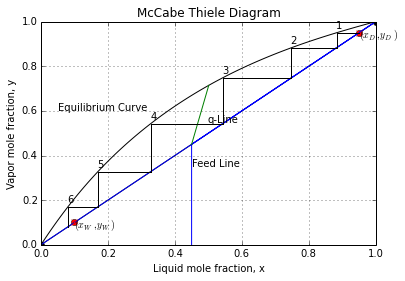## Example 11.4-3 Page Number 662¶

In :
#Number of Trays in Stripping Tower
import matplotlib.pylab as plt
#Variable Declaration
xe = np.array([0.000,0.130,0.258,0.411,0.581,0.780,1.000])
ye = np.array([0.000,0.261,0.456,0.632,0.777,0.900,1.000])
P= 101.3           #Total tower pressure in kPa
F = 400.0          #Feed rate in kmol/hr
xFA = 0.70         #Mole fraction of benzene in feed
xFB = 0.30         #Mole fraction of toulene in feed
W = 60.0           #Bottoms product rate in kmol/hr
xWA = 0.10         #Mole fraction of benzene in bottoms

#Calculations
x = np.arange(0.,1.,0.01)

f = interp1d(xe,ye, kind='cubic')
y = f(x)
plt.title('McCabe Thiele Diagram')
plt.grid(True)
plt.plot(x,y,'k-')
plt.text(.05,0.6, 'Equilibrium Curve')
plt.text(xFA+0.01,xFA-0.1, 'Feed Line')
plt.text(xFA,xFA+0.2, 'q-Line')

plt.plot(xW,xW,'ro')
plt.annotate('$(x_W,y_W)$', xy=(xW,xW), xytext=(xW,xW-0.02))
plt.xlabel('Liquid mole fraction, x')
plt.ylabel('Vapor mole fraction, y')
xWB = 1.- xWA
D = F - W
yDA = (F*xFA-W*xWA)/D
plt.annotate('$(x_F,y_D)$', xy=(xFA,yDA), xytext=(xFA+0.02,yDA+0.02))
plt.plot(xFA,yDA,'ro')
plt.plot([0,1],[0,1])
plt.plot([xFA,xFA],[0,1],'k-')
plt.plot([xFA,xWA],[yDA,xWA],'k-')
plt.plot(xWA,xWA,'bo')
x1 = xFA
y1 = yDA
n = 0
m = (yDA-xWA)/(xFA-xWA)
c = yDA - m*xFA
j = 0
while x1>xW:
y2 = y1
ff = lambda x: y1 -f(x)
sol = root(ff,0.2)
x2 = sol.x
plt.text(x2, y2+0.02, str(n+1))
plt.plot([x1,x2],[y1,y2],'k-')
if x2 > xW:
n = n+1
else:
dxt = x1 - x2
dx = x1 - xW
n = n + dx/dxt
if x2>xW and x2<xF:
j = j + 1

x1 = x2
ff = lambda y: x1 - (y - c)/m
sol = root(ff,0.5)
y2 = sol.x
plt.plot([x1,x2],[y1,y2],'k-')
x1 = x2
y1 = y2
#Results
print "Overhead product rate is", round(D,2),"kmol/hr"
print "Number of equilibrium satges including reboiler for required separation:",round(n,1)
print "Number of equilibrium satges excluding reboiler for required separation:",round(n-1,1)

Overhead product rate is 340.0 kmol/hr
Number of equilibrium satges including reboiler for required separation: 5.3
Number of equilibrium satges excluding reboiler for required separation: 4.3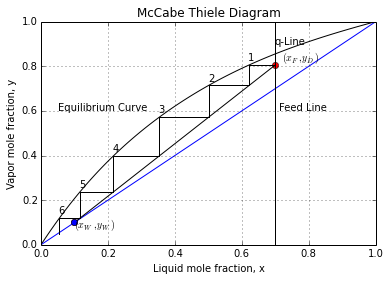## Example 11.6-1 Page Number 670¶

In :
#Enthalpy Concentration Plot for Benzene-Toluene
import numpy as np
import matplotlib.pyplot as plt

#Variable Declaration
xe = np.array([0.000,0.130,0.258,0.411,0.581,0.780,1.000]) #Equillibrium liquid phase composition benzene toulene
ye = np.array([0.000,0.261,0.456,0.632,0.777,0.900,1.000]) #Equillibrium vapor phase composition
T = np.array([110.6,105.0,100.0,95.0,90.0,85.0,80.1])      #Saturation temperatures corresponding to equillibrium points
Tb = np.array([80.1,110.6])      #Boiling point of benzene toulene resp in °C
Cpl = np.array([138.0,167.5])    #Liquid heat capacity of benzene toulene resp kJ/kmol.K
Cpg = np.array([96.3,138.2])     #Vapor heat capacity of benzene toulene resp kJ/kmol.K
Lbd = np.array([30820,33330])    #Latent heat of benzene toulene resp kJ/kmol
h = np.zeros(len(xe))            #Enthalpy of liquid in KJ/kmol
H = np.zeros(len(xe))            #Enthalpy of vapor in KJ/kmol
Tref = 80.1                      #Reference   temperature in °C
#Calculations

LbdA = Cpl*(Tb-Tref) + Lbd - Cpg*(Tb-Tref)
LbdB = Cpl*(Tb-Tref) + Lbd - Cpg*(Tb-Tref)
h = xe*Cpl*(T-Tref)+(1.-xe)*Cpl*(T-Tref)
H = ye*(LbdA + Cpg*(T-Tref)) + (1-ye)*(LbdB + Cpg*(T-Tref))

#Results
print ' Enthalpy (kJ/kgmol) of      '
print '   Liquid     Vapor '
print '     h         H  '
for i in range(len(h)):
print '   %4.0f      %5.0f'%(h[i],H[i])

plt.plot(xe,h,'bo-',ye,H,'ro-')
plt.ylabel('Enthalpy, kJ/kgmol')
plt.xlabel('x, y')
plt.title('Enthalpy concentration Diagram')
plt.grid(True)

 Enthalpy (kJ/kgmol) of
Liquid     Vapor
h         H
5109      38439
4075      36504
3182      35042
2315      33737
1489      32625
708      31653
0      30820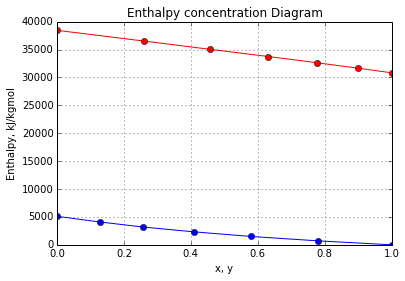## Example 11.6-2 Page Number 674¶

In :
#Distillation Using Enthalpy-Concentration Method
import matplotlib.pylab as plt
import numpy as np
from scipy.interpolate import interp1d
from scipy.optimize import root

#Variable Declaration
#Equillibrium liquid phase composition benzene toulene
xe = np.array([0.000,0.130,0.258,0.411,0.581,0.780,1.000])
#Equillibrium vapor phase composition
ye = np.array([0.000,0.261,0.456,0.632,0.777,0.900,1.000])
#Saturation temperatures corresponding to equillibrium points
T = np.array([110.6,105.0,100.0,95.0,90.0,85.0,80.1])
Tb = np.array([80.1,110.6])      #Boiling point of benzene toulene resp in °C
Cpl = np.array([138.0,167.5])    #Liquid heat capacity of benzene toulene resp kJ/kmol.K
Cpg = np.array([96.3,138.2])     #Vapor heat capacity of benzene toulene resp kJ/kmol.K
Lbd = np.array([30820,33330])    #Latent heat of benzene toulene resp kJ/kmolF = 100.
xF = 0.45           #Feed mole fraction for benzene
xD = 0.95           #Distillate mole fraction for benzene
xW = 0.10           #Bottoms mole fraction for benzene
Rm = 1.17           #Minimum reflux ratio
lambdav = 32099.    #Average Latent heat of vaporiztion kJ/kmol
Cp = 159.           #Average  heat capacity kJ/kmol.K
Tbb = 366.7         #Boiling point of feed in K
Tf = 327.6          #Feed temperature in K
Rmul = 1.5          #Number of times minimum reflux
F = 100.            #Feed rate to distillation, khmol/h

#Calculations
Tref = Tb
LbdA = Cpl*(Tb-Tref) + Lbd - Cpg*(Tb-Tref)
LbdB = Cpl*(Tb-Tref) + Lbd - Cpg*(Tb-Tref)
h = xe*Cpl*(T-Tref)+(1.-xe)*Cpl*(T-Tref)
H = ye*(LbdA + Cpg*(T-Tref)) + (1-ye)*(LbdB + Cpg*(T-Tref))
Hi = interp1d(ye,H,kind='cubic')
hi = interp1d(xe,h,kind='cubic')
yei = interp1d(xe,ye,kind='cubic')

def LiquidH(T,x):
return x*Cpl*(T-Tref)+(1.-x)*Cpl*(T-Tref)

def VaporH(T,y):
return y*(LbdA + Cpg*(T-Tref)) + (1-y)*(LbdB + Cpg*(T-Tref))

R = Rm*Rmul

x = np.arange(0.,1.,0.01)
f = interp1d(xe,ye, kind='cubic')
y = f(x)
plt.title('McCabe Thiele Diagram')
plt.grid(True)
plt.plot(x,y,'k-')
plt.text(.05, .6, 'Equilibrium Curve')
plt.text(xF,xF-0.1, 'Feed Line')
plt.text(xF+0.05,xF+0.1, 'q-Line')
plt.text(xF+0.3,xF+0.3, 'UO Line')
plt.text(xF-0.2,xF-0.2, 'LO Line')
plt.plot(xD,xD,'ro')
plt.plot(xW,xW,'ro')
plt.annotate('$(x_D,y_D)$', xy=(xD,xD), xytext=(xD,xD-0.02))

plt.annotate('$(x_W,y_W)$', xy=(xW,xW), xytext=(xW,xW-0.02))
plt.xlabel('Liquid mole fraction, x')
plt.ylabel('Vapor mole fraction, y')

plt.plot([0,1,xF,xF],[0,1,xF,0])
a = np.array([[1,1], [xD,xW]])
b = np.array([F,F*xF])
[D,W]= np.linalg.solve(a, b)
L = R*D
q = 1+ Cp*(Tbb-Tf)/lambdav
V1 = L + D
H1 = VaporH(82.3,xD)
hD = LiquidH(81.1,xD)
muol = R/(R+1)
cuol = xD/(R+1)
mql = q/(q - 1.)
cql = xF - mql*xF
plt.plot(xD,xD,'ro')
plt.plot(xW,xW,'ro')
a = np.array([[1,-mql], [1,-muol]])
b = np.array([cql,cuol])
[yi,xi] = np.linalg.solve(a, b)
plt.plot([xF,xi],[xF,yi])
xuol = np.arange(0.40,0.96,0.01)
yuol = np.zeros(len(xuol))

m = muol
c = cuol
for j in  range(len(xuol)):
xn = xuol[j]
yn1 = m*xn + c
er = 1.
while er >=0.001:
hn = hi(xn)
Hn1 = Hi(yn1)
Vn1 = (V1*H1-D*hi(xn)+L*hD)/(Hi(yn1)-hi(xn))
Ln = Vn1 - D
yn1c = Ln/Vn1*xn+D*xD/Vn1
er = abs(yn1-yn1c)
yn1 = yn1c
yuol[j] = yn1
plt.plot(xuol,yuol)
Qc = V1*H1-L*hD-D*hD

hF = LiquidH(54.5,xF)
hW = hi(xW)
Qr = D*hD+W*hW+Qc-F*hF

mlol = (xW-yi)/(xW-xi)
clol = yi - mlol*xi
xlol = np.arange(0.1,0.52,0.02)
ylol = np.zeros(len(xlol))
m = mlol
c = clol
yW = yei(xW)
HW = Hi(yW)
hW = hi(xW)
ym1 = yW
Lm = Ln + q*F
Vm1 = Vn1 - (1.-q)*F
for j in  range(len(ylol)):
xm = xlol[j]
ym1 = m*xm - c
er = 1.
while er >=0.001:
hm = hi(xm)
Hm1 = Hi(ym1)
Vm1 = (W*(hm-hW)+Qr)/(Hm1-hm)
Lm = Vm1 + W
ym1c = Lm*xm/Vm1 - W*xW/Vm1
er = abs(ym1-ym1c)
ym1 = ym1c
ylol[j] = ym1c
plt.plot(xlol,ylol)

fl = interp1d(xlol,ylol)
fu = interp1d(xuol,yuol)

ff = lambda x: f(x)-(mql*x+cql)
sol = root(ff,0.52)
xq = sol.x
yq = f(xq)

x1 = xD
y1 = xD
n = 0
j = 0
while x1>xW:
y2 = y1
ff = lambda x: y1 -f(x)
sol = root(ff,0.2)
x2 = sol.x
plt.text(x2-0.02, y2+0.02, str(n+1))
plt.plot([x1,x2],[y1,y2],'k-')
if x2 > xW:
n = n+1
else:
dxt = x1 - x2
dx = x1 - xW
n = n + dx/dxt

if x2>xW and x2<xi:
j = j + 1

x1 = x2
if x1 >= xi:
ff = lambda y: y - fu(x1)
sol = root(ff,0.65)
y2 = sol.x
elif x1 <= xi and x1 >=xW:
ff = lambda y: y - fl(x1)
sol = root(ff,0.2)
y2 = sol.x
else:
y2 = x1

plt.plot([x1,x2],[y1,y2],'k-')
x1 = x2
y1 = y2

#Results
print 'Vapor rate from reboiler %4.1f kmol/h'%Vm1
print 'Bottoms product rate %4.1f kJ/h'%W
print 'Distillate rate %4.1f kJ/h'%D
print 'Condeser duty %8.2f kJ/h'%Qc
print 'Reboiler duty %8.2f kJ/h'%Qr
print "Number of equilibrium stages including reboiler for required separation:",round(n,1)
print "Number of equilibrium stages excluding reboiler for required separation:",round(n-1,1)
print 'Feed is introduced on %2d'%(ceil(n-j))

Vapor rate from reboiler 128.2 kmol/h
Bottoms product rate 58.8 kJ/h
Distillate rate 41.2 kJ/h
Condeser duty 3524297.05 kJ/h
Reboiler duty 4178128.14 kJ/h
Number of equilibrium stages including reboiler for required separation: 10.9
Number of equilibrium stages excluding reboiler for required separation: 9.9
Feed is introduced on  6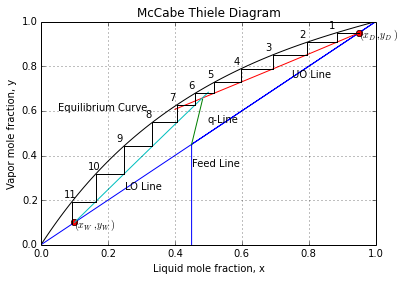## Example 11.7-1 Page Number 682¶

In :
#Boiling Point of Multi-Component Liquid
import numpy as np

#Variable Declaration
x = np.array([0.40,0.25,0.20,0.15])       #Feed composition in molfrac
K1 = np.array([1.68,0.630,0.245,0.093])   #Values at 65 °C are taken from Fig 11.7-2
K2 = np.array([1.86,0.710,0.2815,0.110])  #Values at 70 °C are taken from Fig 11.7-2

#Calcualtion
k = 2
alpha = K1/K1[k]
alpx = alpha*x
s1 = s2 = 0.0
for j in range(len(x)):
s1 = s1 + alpx[j]

Kc = 1./s1
print "Kc:", round(Kc,4), "This value corresponds to 69°C "

alpha = K2/K2[k]
alpx = alpha*x
print "At 69°C the value calculated is not shown and corresponds to 70°C"
for j in range(len(x)):
s2 = s2 + alpx[j]

Kc = 1./s2

#Results
print "Kc:", round(Kc,4), "This value corresponds to 70°C Hence converged"
print "Bubble temperature is equal to 70 °C "

Kc: 0.2745 This value corresponds to 69°C
At 69°C the value calculated is not shown and corresponds to 70°C
Kc: 0.2831 This value corresponds to 70°C Hence converged
Bubble temperature is equal to 70 °C


## Example 11.7-2 Page Number 684¶

In :
#Calculation of Top and Bottom Temeperature and Total Reflux
import numpy as np
from scipy.optimize import root

#Variable Declaration
Name = np.array(['A','B','C','D'])              #Component Nomenclature C4,C5,C6,C7
xF = np.array([0.40,0.25,0.20,0.15])            #Feed composition in molfrac

xW = np.array([0.0011,0.0704,0.5068,0.4217])    #Bottoms composition in molfrac
K993 = np.array([3.12,1.38,0.60,0.28])          #Values of K at 99.3 °C
K670 = np.array([1.75,0.65,0.26,0.10])          #Values of K at 67.0 °C for first trial
K132 = np.array([5.00,2.35,1.15,0.61])          #Values of K at 132.0 °C for first trial
yD = np.zeros(4)
xW = np.zeros(4)

F = 100.            #Molar feed rate to column, mol/h
xBD = 0.90          #Fraction of B in Distillate
xCW = 0.90          #Fraction of C in Bottoms
hk = 2              #Heavy key index
lk = 1              #Light key index
q = 1.0             #Value of q as feed is saturated

#Calculations
mBD = F*xF[lk]*xBD
mBW = F*xF[lk]-mBD
mCW = F*xF[hk]*xCW
mCD = F*xF[hk]-mCW

#With assumption of no moles of D in Distillate and no moles of A in Bottoms
mDW = F*xF

#Calculate Flow rate of Distillate and Bottoms
W = mBW+mCW+mDW
yD = mBD/D
yD = mCD/D
xW = mBW/W
xW = mCW/W
xW = mDW/W

#Dew Point Calculations for Distillate with initial guess of 67°C
alp67 = K670/K670[hk]
alphalkD = alp67[lk]

ybyalp = yD/alp67
Syba = 0.0

for j in range(len(yD)):
Syba = Syba + ybyalp[j]

x = ybyalp/Syba
Sx = 0.0
for j in range(len(xW)):
Sx = Sx + x[j]

#Bubble Point Calculations for Bottoms with initial guess of 132°C
alp132 = K132/K132[hk]
alpixi = xW*alp132
Sxa = 0.0
for j in range(len(yD)):
Sxa = Sxa + alpixi[j]

y = alpixi/Sxa
Sy = 0.0
for j in range(len(yD)):
Sy = Sy + y[j]

alphalkW = alp132[lk]

alpavg = sqrt(alphalkD*alphalkW)

Nm = log((yD[lk]/yD[hk])*(xW[hk]/xW[lk]))/log(alpavg)
alpavA = sqrt(alp67*alp132)           #Average relative volatility of Butane
alpavD = sqrt(alp67*alp132)           #Average relative volatility of Butane

#Distribution of Componenet in Distillate and Bottoms

DbyWA = alpavA**Nm*yD[hk]*D/(xW[hk]*W)
DbyWD = alpavD**Nm*yD[hk]*D/(xW[hk]*W)

mAW = xF*F/(1.0+DbyWA)
mDW = xF*F/(1.0+DbyWD)
mDD = mDW*DbyWD

#Revised Distillate and Bottoms Compositions
W = mAW+mBW+mCW+mDW
yD = mBD/D
yD = mCD/D
yD = mDD/D

xW = mAW/W
xW = mBW/W
xW = mCW/W
xW = mDW/W

#Dew Point Calculations for Distillate with revised compositions
alphalkD = alp67[lk]

ybyalp = yD/alp67
Syba = 0.0

for j in range(len(yD)):
Syba = Syba + ybyalp[j]

x = ybyalp/Syba
Sx = 0.0
for j in range(len(xW)):
Sx = Sx + x[j]

#Bubble Point Calculations for Bottoms with revised compositions
alpixi = xW*alp132
Sxa = 0.0
for j in range(len(yD)):
Sxa = Sxa + alpixi[j]

y = alpixi/Sxa
Sy = 0.0
for j in range(len(yD)):
Sy = Sy + y[j]

#Results
print "For Part A"
print "Distillate Compositions are:"
for i in range(len(yD)):
print Name[i],":",round(yD[i],4)
print "Bottoms Compositions are:"
for i in range(len(xW)):
print Name[i],":",round(xW[i],4)
print "Distillate and Bottoms rate are", round(D,3), "and",round(W,3)

print "For Part B"
print "Dew temperature for top product and Bubble temperature for Bottoms are:\nguess values of 67 and 132 °C"

print "For Part C"
print "Minimum number of stages including reboiler are:", round(Nm,3)
print "Minimum number of stages excluding reboiler are:", round(Nm-1,3)

For Part A
Distillate Compositions are:
A : 0.6197
B : 0.3489
C : 0.031
D : 0.0004
Bottoms Compositions are:
A : 0.0011
B : 0.0704
C : 0.5068
D : 0.4217
Distillate and Bottoms rate are 64.483 and 35.517
For Part B
Dew temperature for top product and Bubble temperature for Bottoms are:
guess values of 67 and 132 °C
For Part C
Minimum number of stages including reboiler are: 5.389
Minimum number of stages excluding reboiler are: 4.389


## Example 11.7-3 Page Number 687¶

In :
#Minimum Reflux and Number of Stages at Operating Reflux
import numpy as np
from scipy.optimize import root

#Variable Declaration
xF = np.array([0.40,0.25,0.20,0.15])            #Feed composition in molfrac
xD = np.array([0.6197,0.3489,0.0310,0.0004])    #Distillate composition in molfrac
xW = np.array([0.0011,0.0704,0.5068,0.4217])    #Bottoms composition in molfrac
K = np.array([3.12,1.38,0.60,0.28])             #Values of K at 99.3 °C

hk = 2                              #Heavy key index
lk = 1                              #Light key index
q = 1.0                             #Value of q as feed is saturated
alavlk,D,W = 2.258,64.484,35.516    #From Example 11.7-2
#Calculation
al = K/K[hk]
alxF = al*xF
alxD = al*xD
f = lambda x: alxF/(al-x)+ alxF/(al-x)+ alxF/(al-x)+ alxF/(al-x)
sol = root(f,1.1)
theta = sol.x
Rm = alxD/(al-theta)+ alxD/(al-theta)+ alxD/(al-theta)+ alxD/(al-theta) - 1.0
R = 1.5*Rm
ordiR = R/(1+R)
Param = Rm/(1+Rm)
#From Fig 11.7-3
Nm = log((xD[lk]/xD[hk])*(xW[hk]/xW[lk]))/log(alavlk)
NmbyN = 0.49
N = Nm/NmbyN
ff = lambda Ne:log(Ne/(N-Ne))-0.206*log((xF[hk]/xF[lk])*(W/D)*(xW[lk]/xD[hk]))
sol = root(ff,5)
Ne = sol.x
#Results
print "For Part A "
print "Minimum reflux is",round(Rm,3)
print "For Part B"
print "Number of Theoretical Stages including reboiler are", round(N,1)
print "Number of Theoretical Stages excluding reboiler are", round(N-1,1)
print "For Part C"
print "Feed is introduce on tray number", round(Ne,0),"from top"

For Part A
Minimum reflux is 0.395
For Part B
Number of Theoretical Stages including reboiler are 11.0
Number of Theoretical Stages excluding reboiler are 10.0
For Part C
Feed is introduce on tray number 6.0 from top